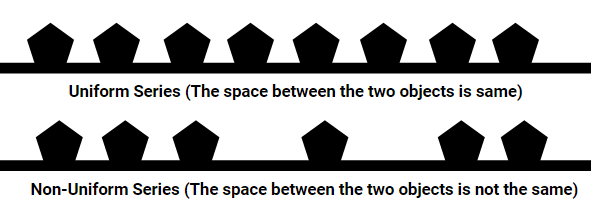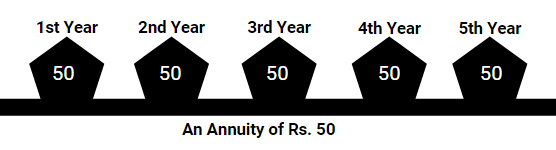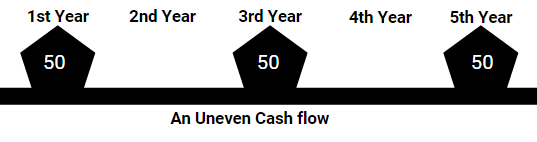# Future Value of a Series of Cash Flow

Future Value of a Series of Cash Flow is used to determine the total worth of continuous cash flow of some years at a prevalent rate of interest. In simple words, it is the total return to be obtained after the maturity of a continuous deposit scheme in the money was invested at regular intervals. E.g. The total return on a bank recurring deposit in which money was invested uniformly for 5 years.

### Uniform series and Non-Uniform series of payments

• The word series means something one after the other in a pattern, like weekly episodes of a daily soap, 5 volumes of a book, etc.
• If the episodes of a daily soap are telecasted weekly, then it is known as uniform series and the duration between two episodes is the same.
• The duration between the volume of two books may or may not be the same if it is different it is known as non-uniform series.• An Annuity is a uniform series of payments made at equal intervals. Examples of Annuity include recurring deposit account, loan repayment in fixed EMIs, etc.• If the amount paid is variable at intervals or different amount is paid at the same intervals, then it is called as uneven cash flow instead of Annuity.#### Example 1:

Sanjay invests ₹25000 every year in mutual funds and Rakesh pays ₹15000 every month as loan EMI are examples of an annuity.

### Types of Annuities (Annuity Due, Ordinary Annuity and Perpetuity)

• Annuity Due: In this Annuity, the amount is paid at the beginning of each period.
• Ordinary Annuity: In this Annuity, the amount is paid at the end of each period.
• Perpetuity: This is an Annuity for the Infinite Period.

#### Example. 2:

• Ruchi pays her LIC premium of Rs 10000 at the beginning of each year is an Annuity Due
• Nisha deposits Rs 500 in her recurring deposit account at the end of every month is an Ordinary Annuity
• Dividend paid by a company on its share for a lifetime, hence it’s a Perpetuity
• In case of an Annuity Due, as the installment is paid at the beginning of the year, hence all installments are considered for interest payments.
• In the case of Ordinary Annuity, the installment is paid at the end of the year, hence the last installment is not considered for interest calculation.

### Examples and Formula for Future Value of Cash Flow

#### Example 3.

• Abhishek has deposited Rs. 10000 in a bank that offers 10% interest. Calculate the Future value of Abhishek's investment after 2 years.
• Solution:  The formula for Future Value in Single cash flow is FV = P (1+R)
• Where P is the amount invested at R% per year for T years
• Hence, FV = 10,000 (1 + 0.1)2 = Rs. 12,100.00
• The Future Value of an ordinary annuity and annuity due is calculated by adding future values of individual installments as shown below:
• The formula to calculate the future value of a single installment is the same as that of compound interest: FV = P (1+R)T

#### Example 4:

• Consider an ordinary annuity of ₹10000 for 5 years at the rate of 10% PA compounded annually.
Years Completed 0 1 2 3 4 5
Instalment Paid 0 ₹10000 ₹10000 ₹10000 ₹10000 ₹10000
Number of years for which interest will be calculated on an installment (T) 5 (5-0) 4 (5-1) 3 (5-2) 2 (5-3) 1 (5-4) 0 (5-5)
Calculation of Amount (Using FV of single cash flow)  0 ₹ 14,641.00   ₹ 13,310.00   ₹ 12,100.00   ₹ 11,000.00   ₹ 10000
• Here, the Future Value will be the sum of future values of all payments made, i.e. FV = Rs 60871.00
• Instead of following this lengthy process, there is a direct formula to calculate the future value of a series of cash flow:
• Future Value of Ordinary Annuity: FV = A {[1 + r]N – 1/r}, where A Is the amount paid per period for N years and r is the rate of interest

#### Example 5:

• Sanjay invests Rs 25000 at the end of every year in a deposit account for 2 years, the expected rate of return is 10%. Sanjay wants to know what the Future Value of his investments will be, let us help him.
• Solution: As Sanjay deposits money at the end of every year, it is a case of an ordinary annuity,
• A = 25000, r = 0.1 and N =2, substituting the values in formula obtained above,
• FV = 25000 [(1 + 0.1)2-1/0.1]  = Rs 52500.00

#### Example 6:

• Consider an annuity due to Rs 10000 for 5 years at the rate of 10% PA compounded annually.
Years Completed 0 1 2 3 4 5
Instalment Paid Rs 10000 Rs 10000 Rs 10000 Rs 10000 Rs 10000 0
Number of years for which interest will be calculated on an installment (T) 5 (5-0) 4 (5-1) 3 (5-2) 2 (5-3) 1 (5-4) 0 (5-5)
Calculation of Amount (Using FV of single cash flow) Rs 16105.00   Rs 14,641.00   Rs 13,310.00   Rs 12,100.00   Rs 11,000.00   0
• The Future Value will be some of the future values of all payments made. Hence, FV = Rs 67156.00
• Instead of following this lengthy process, there is a direct formula to calculate the future value of a series of cash flow
• Future Value of Annuity Due: FV = A {[1 + r]N – 1/r} (1+r), where A Is the amount paid per period for N years and r is the rate of interest.
• In case of uneven cash flows, there cannot be any standard formula as the installment amount keeps changing, therefore it is advised to calculate Future Value for uneven cash flows by adding future values of individual installments.

#### Example. 7:

• Ram deposits Rs 2000 for six months in his savings account, but after six months his expense increase so now, he deposits Rs 1000 every month for the remaining months of the Year.